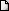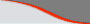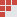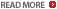MBA Entrance exams in India - CAT, XAT entrance exam sample practice problems - Set TheoryLATEST NEWS • Ascent Success Stories • TANCET 16 Classes @ Chennai • XAT 16 Classes @ ChennaiHome | TANCET Coaching Class | TANCET study material | Online TANCET Course | Contact us
 Ascent's Courses TANCET Coaching Chennai Online TANCET Course TANCET MBA Study Material CAT Classroom Chennai CAT Correspondence India Mock CAT Series GRE Coaching Chennai GMAT Coaching Chennai Online GMAT CourseUS B Schools Faculty List Jobs @ Ascent Testimonials Ascent Success Stories Contact Us +91 44 4500 8484 +91 96000 48484

### Set Theory - Quant/Math - CAT 2016

Question 4 the day: August 3, 2006

The CAT Practice question for the day is from the topic Set Theory.

Question
In a class of 120 students numbered 1 to 120, all even numbered students opt for Physics, whose numbers are divisible by 5 opt for Chemistry and those whose numbers are divisible by 7 opt for Math. How many opt for none of the three subjects?
1. 19
2. 41
3. 21
4. 26
Correct choice (2). Correct Answer - 41

We need to find out the number of students who took at least one of the three subjects and subtract that number from the overall 120 to get the number of students who did not opt for any of the three subjects.

Number of students who took at least one of the three subjects can be found by finding out A U B U C, where A is the set of those who took Physics, B the set of those who took Chemistry and C the set of those who opted for Math.

Now, AUBUC = A + B + C - (A n B + B n C + C n A) + (A n B n C)
A is the set of those who opted for Physics = 120/2 = 60 students
B is the set of those who opted for Chemistry = 120/5 = 24
C is the set of those who opted for Math = 120/7 = 17.

The 10th, 20th, 30th numbered students would have opted for both Physics and Chemistry.
Therefore, A n B = 120/10 = 12

The 14th, 28th, 42nd. Numbered students would have opted for Physics and Math.
Therefore, C n A = 120/14 = 8

The 35th, 70th . Numbered students would have opted for Chemistry and Math.
Therefore, A n B = 120/35 = 3

And the 70th numbered student would have opted for all three subjects.

Therefore, AUBUC = 60 + 24 + 17 - (12 + 8 + 3) + 1 = 79.

Number of students who opted for none of the three subjects = 120 - 79 = 41.

## XLRI XAT TANCET Practice Question Samples

TANCET Questionbank
 TANCET MBA MCA Practice Questions with detailed explanation and shortcuts.GMAT Math Books
 Comprehensive study material for GMAT Quant. 1800+ questions.CAT Online Course

 GMAT Diagnostic Test

 CAT Discussion Forum

 GMAT Math Lesson Book | SAT prep | GRE Classes @ Chennai | GMAT Coaching @ Chennai | TANCET Mailing List © 2002 - 17 ASCENT Education all rights reserved. Terms & Condition | Privacy Policy